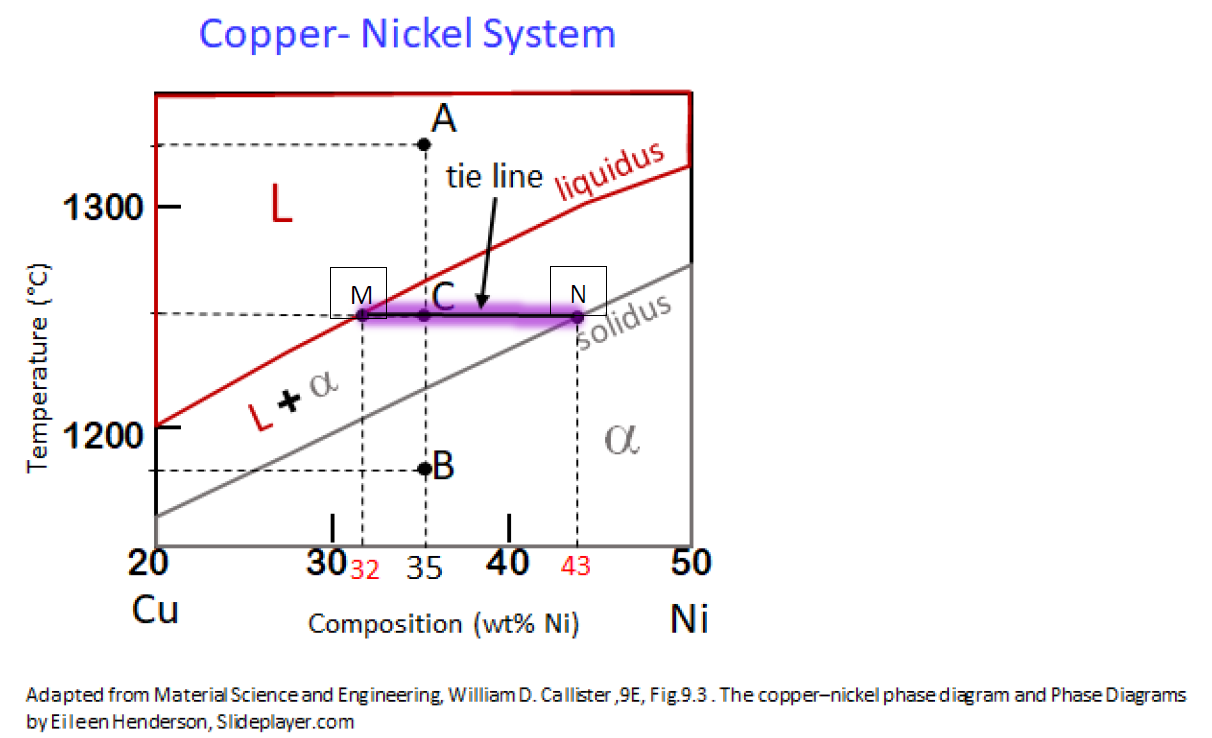### Gibbs Phase rule

Gibbs Phase rule
It expresses mathematically the general relationship for the existence of stable phases corresponding to the equilibrium conditions.  The Phase rule derived from thermodynamics state that
C + N = P + F
Where,
P:  Phases at given composition
F: DoF (Degrees of freedom) i.e., temperature, composition, pressure
C: How many Components
N: How many variables (Non compositional)

Gibbs Phase rule determines the number of independent variables that have to be defined to be able to determine state of a system.
Example: Copper- Silver phase diagram
Let us take an example of the copper-silver phase diagram.
The red spot is indicated in the figure . With respect to the red spot on the figure,let us substitute the values in the Gibbs equation. Pressure (1atm)

Parameter:
N =1(temperature parameter), C=2 (Copper and Ag), P=one single phase
Substituting the values of non-compositional variables number of components number of faces present,
We get,
F = 2+1-1 = 2
The degrees of freedom obtained is 2.  This indicates that to determine the characteristics at the given point on the phase diagram, minimum two variables are required with respect to the example given the two variables are temperature and pressure.

Other rules governing phase diagram
The Lever Rule

• Rule 1: If we know Temperature and Composition (Co), then phases present can be known.Consider point A & B from the copper Nickel phase diagram.  If we need to determine the Phase at the point A & B then according to rule 1, by knowing the temperature and composition we can say that point A is in the liquid phase and point B is in solid phase.

• Rule 2: If Temperature and Composition (Co) are known, the composition of each phase can be found.
Consider the same point A & B from the copper Nickel phase diagram. If we need to determine the phase composition at that point A & B then according to rule 2, by knowing the temperature and overall composition we can say that at point A & B, the composition of liquid and solid is equal to its overall composition respectively.
i.e., CL = Cα = CO = 35wt % of Ni and 65 wt % of Cu

• Rule 3: If Temperature and Composition (Co) are known, then the amount of each phase can be found.
Consider the same point A & B from the copper Nickel phase diagram. If we need to determine the amount of each phase at the point A & B then according to rule 3, by knowing the temperature and overall composition we can say that at point A & B, the amount of liquid and solid is equal to its overall composition respectively.
i.e., WL = WO = 100 wt %  whereas  Wα = 0 at Point A  &  Wα = WO = 100 wt %   whereas WL = 0 at Point B

Let us consider point C and implement all three rules.
The point C lies in a region between solidus and liquidus lines. There exists both liquid as well as solid phases. With respect to rule 1, the number of phases present is 2.
To determine the composition of liquid face and composition of solid phase first we will have to draw a tie line which is a horizontal line drawn from the point C and this line should intersect the liquidus line at point M and solidus line at N. Now drop vertical lines from the point M and N, wherever this vertical line meet the x-axis will be the composition of the liquid phase and solid phase.
CL = CLiquidus = 32 wt % Ni
Cα  = CSolidus   = 43 wt % Ni

In the diagram above we have drawn a tie line MN passing through the point C. If we start from N and make a way towards C we can observe that at point N the alloy is completely solid and it starts converting to liquid as we go along the horizontal tie line towards liquidus and at point M the alloy would have completely converted to liquid. So at point C, NC part of the tie line would have already converted to liquid and NM part of the tie line would still be a solid. Therefore to find the amount of liquid phase,
Wα = CM/MN  ×100
i.e.   Wα = (Co-CL)/(C∝-CL )  ×100
Similarly,
WL = NM/MN  ×100
i.e. WL = (C∝-Co)/(C∝-CL )  ×100